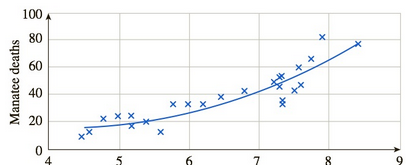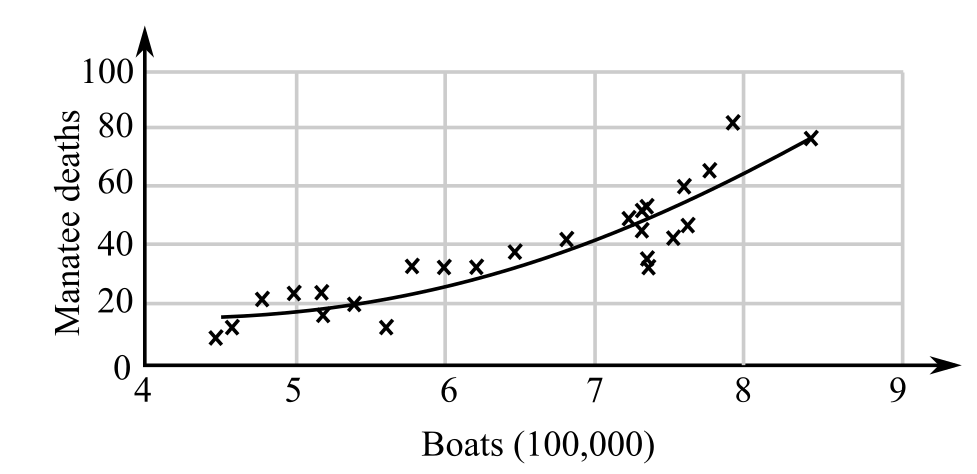Chapter 10.6, Problem 51EFinite Mathematics and Applied Cal...

7th Edition
Stefan Waner + 1 other
ISBN: 9781337274203

Solutions

Chapter
SectionFinite Mathematics and Applied Cal...

7th Edition
Stefan Waner + 1 other
ISBN: 9781337274203
Textbook Problem

Ecology Increasing numbers of manatees have been killed by boats off the Florida coast. The following graph shows the relationship between the number of boats registered in Florida and the number of manatees killed each yearBoats (100,000)The regression curve shown is given by f ( x ) = 3.55 x 2 − 30.2 x + 81  manatee deaths                                                          ( 4.5 ≤ x ≤ 8.5 ) where x is the number of boats (hundreds of thousands) registered in Florida in a particular year and f ( x ) is the number of manatees killed by boats in Florida that year.80 Compute and interpret f ′ ( 8 ) .

To determine

To calculate: The value of f(8)

for the function f(x)=3.55x230.2x+81, where x represents the number of boats (hundreds of thousands) registered in Florida in a particular year and f(x)

represents the number of manatees killed by boats in Florida that year, also interpret the answer and the graph which shows the relation between the numbers of boats registered in Florida and the number of manatees killed each year is as follows:Explanation

Given information:

The function f(x)=3.55x230.2x+81, where x represents the number of boats (hundreds of thousands) registered in Florida in a particular year and f(x)

represents the number of manatees killed by boats in Florida that year.

The graph which shows the relation between the numbers of boats registered in Florida and the number of manatees killed each year is as follows:

Formula used:

The difference quotient formula is used which is as follows:

f(x)=limh0f(x+h)f(x)h

The square of sum formula as,

(a+b)2=a2+b2+2ab

Calculation:

Consider the function f(x)=3.55x230.2x+81, where x represents the number of boats (hundreds of thousands) registered in Florida in a particular year and f(x)

represents the number of manatees killed by boats in Florida that year.

The difference quotient formula used to estimate the exact value of derivative algebraically is:

f(a)=limh0f(a+h)f(a)h.

Substitute a=8

into the above formula,

f(8)=limh0f(8+h)f(8)h

Substitute the values of f(8)

and f(8+h)

in f(x),

f(8)=limh0[3

Still sussing out bartleby?

Check out a sample textbook solution.

See a sample solution

The Solution to Your Study Problems

Bartleby provides explanations to thousands of textbook problems written by our experts, many with advanced degrees!

Get Started

(a) Do matrices A and B have the same order? (b) Does matrix A equal matrix B? (c) Does BT = A?

Mathematical Applications for the Management, Life, and Social Sciences

True or False: is a convergent series.

Study Guide for Stewart's Multivariable Calculus, 8th

Simplified, log3 9x3 is: 2 + 3 log3 x 6 log3 x 9 log3 x 9 + log3 x

Study Guide for Stewart's Single Variable Calculus: Early Transcendentals, 8th